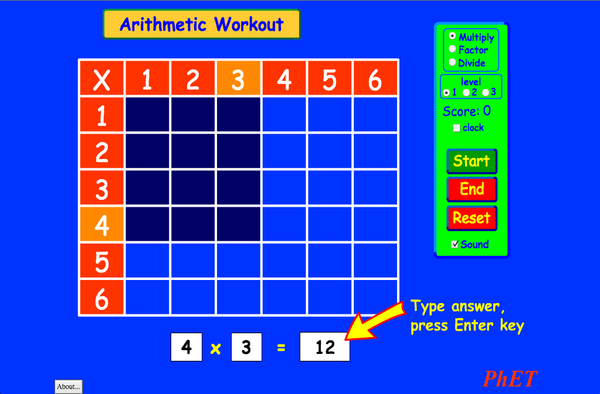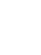# अंकगणितउतरवून घ्याअंतःस्थापित बंद या सादृश्याची प्रत अंतःस्थापित करून घ्या. या सादृश्याची प्रत अंतःस्थापित करून घेण्यासाठी हे HTML वापरा.उंची आणि रुंदी याची परीमाणे बदलून अंतःस्थापित केलेल्या सादृश्याची उंची आणि रुंदी तुम्हाला बदलता येईल. Embed an image that will launch the simulation when clicked
सुरु करण्यासाठी क्लिक करा.
"सुरु करण्यासाठी क्लिक करा" यावाक्यासह चित्रझलक दिसण्यासाठी हा HTML संच वापरा. गुणन तसेच तुमच्यासारखे सल्लागारPhET ला सहकार्य करतात.Back to HTML5 Version

• गुणन

### Description

Remember your multiplication tables? Practice your multiplication, division, and factoring skills with this exciting game. No calculators allowed!

### नमुना शैक्षणिक उद्दिष्ट्ये

• Explain how multiplication tables help understand multiplication, factoring, and division.
• Use an array model to understand multiplication, factoring, and division.
• Increase accuracy in multiplying, factoring and dividing.
• Develop multiple strategies for arithmetic problems.

### Standards Alignment

#### Common Core - Math

3.OA.A.1
Interpret products of whole numbers, e.g., interpret 5 × 7 as the total number of objects in 5 groups of 7 objects each. For example, describe a context in which a total number of objects can be expressed as 5 × 7.
3.OA.A.2
Interpret whole-number quotients of whole numbers, e.g., interpret 56 ÷ 8 as the number of objects in each share when 56 objects are partitioned equally into 8 shares, or as a number of shares when 56 objects are partitioned into equal shares of 8 objects each. For example, describe a context in which a number of shares or a number of groups can be expressed as 56 ÷ 8.
3.OA.A.4
Determine the unknown whole number in a multiplication or division equation relating three whole numbers. For example, determine the unknown number that makes the equation true in each of the equations 8 × ? = 48, 5 = _ ÷ 3, 6 × 6 = ?
3.OA.B.5
Apply properties of operations as strategies to multiply and divide.2 Examples: If 6 × 4 = 24 is known, then 4 × 6 = 24 is also known. (Commutative property of multiplication.) 3 × 5 × 2 can be found by 3 × 5 = 15, then 15 × 2 = 30, or by 5 × 2 = 10, then 3 × 10 = 30. (Associative property of multiplication.) Knowing that 8 × 5 = 40 and 8 × 2 = 16, one can find 8 × 7 as 8 × (5 + 2) = (8 × 5) + (8 × 2) = 40 + 16 = 56. (Distributive property.)
3.OA.B.6
Understand division as an unknown-factor problem. For example, find 32 ÷ 8 by finding the number that makes 32 when multiplied by 8.
3.OA.C.7
Fluently multiply and divide within 100, using strategies such as the relationship between multiplication and division (e.g., knowing that 8 × 5 = 40, one knows 40 ÷ 5 = 8) or properties of operations. By the end of Grade 3, know from memory all products of two one-digit numbers.
3.OA.D.9
Identify arithmetic patterns (including patterns in the addition table or multiplication table), and explain them using properties of operations. For example, observe that 4 times a number is always even, and explain why 4 times a number can be decomposed into two equal addends.
Version 2.03

### Teacher Tipsteacher's guide (pdf) मध्ये PhET चमूने तयार केलेल्या उपयुक्त सुचना आहेत. ( PDF ).

### Teacher-Submitted Activities

Juego de Aritmética (Basado en Indagación)Trish Loeblein, traducido por Diana López माध्य.
उच्च
HW
Guided
गणित
How do PhET simulations fit in my middle school program?Sarah Borenstein माध्य. इतर रसायनशास्त्र
जीवशास्त्र
भौतिक्शास्त्र
भू विज्ञान
MS and HS TEK to Sim Alignment Elyse Zimmer उच्च
माध्य.
इतर भौतिक्शास्त्र
रसायनशास्त्र
जीवशास्त्र
Math facts - Using the Multiplication Chart Tapan Sarkar माध्य. प्र.शाळा गणित
Back to the Basics Nyetta Abernathy प्राथ.
उच्च
माध्य.
HW
प्र.शाळा
गणित
Discovering divisibility rules Stacy Larson उच्च
माध्य.
प्र.शाळा गणित
곱셈 나눗셈 연습 SIM 사용설명서 이화국(Wha Kuk Lee) माध्य.
प्राथ.
HW
CQs
गृहपाठ
गणित
भौतिक्शास्त्र
ARITHMETIC_DIVISON Arlete Cabral प्राथ. प्र.शाळा गणित
OBJETO DE APRENDIZAGEM: ARITHMETIC Arlete Moura de Oliveira Cabral e Maria Cleide da Silva Barroso प्राथ. Guided गणित
Operações Aritméticas Fundamentais Georgyana Cidrão; Francisco Régis Vieira Alves प्राथ. प्र.शाळा
Guided
गणित
भाषा Downloador RunTips
Afrikaans All AfrikaansRekenkunde
Albanian All shqipAritmetika
Amharic All Amharicየሒሳብ ስሌቶች
Arabic All العربيةarithmetic
Arabic, Saudi Arabia All العربية (السعودية)arithmetic
Basque All EuskaraAritmetika
Bosnian All BosanskiARITMETIKA
Brazilian Portuguese All português (Brasil)Aritmética
Chinese - Simplified All 中文 (中国)算术
Chinese - Traditional All 中文 (台灣)算數
Croatian All hrvatskiAritmetika
Danish All DanskAritmetisk
Dutch All NederlandsRekenen
Estonian All EestiAritmeetika
French All françaisArithmétique
Georgian All ქართულიარითმეტიკა
German All DeutschRechnen
Greek All ΕλληνικάΑριθμητικός
Hebrew All עבריתחשבון
Hungarian All magyarSzorzótábla játék
Indonesian All Bahasa IndonesiaAritmatika
Italian All italianotabelline
Japanese All 日本語計算
Kazakh All KazakhАрифметика
Korean All 한국어곱셈 나눗셈 연습
Latvian All LatviešuAritmētika
Macedonian All македонскиАРИТМЕТИКА
Persian All فارسیحساب
Polish All polskiArytmetyka
Romanian All românăAritmetica
Russian All русскийАрифметика
Serbian All СрпскиАРИТМЕТИКА
Slovak All SlovenčinaAritmetika
Spanish All españolAritmética
Spanish (Mexico) All español (México)Aritmética
Spanish (Peru) All español (Perú)Aritmética
Swahili All SwahiliHesabu
Swedish All svenskaMatteräkning
Turkish All TürkçeAritmetik
Turkmen All TurkmenArifmetika
Vietnamese All Tiếng ViệtSố học
इंग्रजी All EnglishArithmetic
Windows Macintosh Linux
Microsoft Windows
XP/Vista/7/8.1/10
Macromedia Flash 9 or later
OS X 10.9.5 or later
Macromedia Flash 9 or later
Macromedia Flash 9 or later
संकल्पना संघ तृतीयपक्षी संदर्भ यांचे आभार